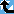# Euler Irregular primes

The Prime Pages keeps a list of the 5000 largest known primes, plus a few each of certain selected archivable forms and classes. These forms are defined in this collection's home page.

Kummer proved the first case of Fermat's Last Theorem for every prime $p$ that does not divide the numerator of any Bernoulli number $B(2n)$ with $0 \lt 2n \lt p-1.$ Vandiver likewise proved it for Euler-regular primes [Vandiver1940]. (See also http://groups.yahoo.com/group/primenumbers/message/4197.)

Definition (Vandiver): A prime $p$ is Euler-irregular (E-irregular) if and only if it divides an Euler number $E(2n)$ with $0 \lt 2n \lt p-1.$

Euler numbers are obtained from Taylor coefficients of

$$\frac{1}{\cosh x} = \sum_{k \ge 0} \frac{E(k) x^k}{k!}$$ giving $$E(0) = 1,\, E(2) = -1,\, E(4) =5,\, E(6) = -61,\, E(8) = 1385 ...$$ The smallest E-irregular prime is $p = 19,$ which divides $E(10) = - 50521.$ The first few E-irregular primes are
19, 31, 43, 47, 61, 67, 71, 79, 101, 137, 139, 149, 193, 223, 241,
with $p = 241$ dividing both $E(210)$ and $E(238)$ hence having an E-irregularity index of 2.

Vandiver proved [Vandiver1940] that $x^p+y^p= z^p$ has no solution for integers $x,$ $y,$ $z$ with $\gcd(xyz,p) = 1$ if $p$ is Euler-regular. Gut proved [Gut1950] that $x^{2p}+y^{2p}= z^{2p}$ has no solution if $p$ has an E-irregularity index less than 5.

It was proven in [Carlitz1954] that there is an infinity of E-irregular primes. In [Ernvall1975] a stronger result was obtained: there is an infinity of E-irregular primes with residue 1 modulo 8. As in the case of Kummer's B-regular primes, there is as yet no proof that there are infinitely many E-regular primes, though this seems likely to be true.

Like B-irregularity, E-irregularity relates to the divisibility of class numbers of cyclotomic fields [Ernvall1979]. In the case of Kummer's B-irregularity one is working with the trivial character $\chi(k) = 1$ of the Riemann zeta function

$$\zeta(n) = \sum_{k \ge 0} \frac{1}{k^n}$$ which evaluates to $$\zeta(2n) = - (-4\pi^2)^n\frac{B(2n)}{2(2n)!}$$ at the even positive integers. E-irregularity entails the unique character modulo 4, namely $\chi(k)=\sin(k\pi/2)$, with the corresponding Dirichlet series $$\beta(n) = \sum_{k \gt 0}{\frac{\sin(k\pi/2)}{k^n}}$$ evaluating to $$\beta(2n+1) = \pi \left(-\frac{\pi^2}{4}\right)^{\!\!n} \frac{E(2n)}{4(2n)!}$$

at the odd positive integers, with Gregory's formula

$$\frac{\pi}{4} = 1 - \frac{1}{3} + \frac{1}{5} - \frac{1}{7} + \frac{1}{9} - \frac{1}{11} + \cdots$$

being the first example. In [Ernvall1983] it was proven that for every primitive character there is an infinite number of corresponding generalized irregular primes. It is conjectured that the probability of a random prime having E-irregularity index $k$ is given by the Poisson distribution

Conjecture: $P(k) = \displaystyle\frac{e^{-1/2}}{2^k k!}$
and hence that a fraction $1-e^{-1/2}$ (about 39.35%) of primes are E-irregular, as is also conjectured in the case of Kummer's B-irregular primes.

The E-irregular primes less than 10,000 were found in [EM1978], using modular arithmetic to determine divisibility properties of the corresponding Euler numbers. I have re-computed the irregular pairs $(p,2n)$ with $0 \lt 2n \lt p-1 \lt 10,000$ and $E(2n)=0 \pmod{p}.$ The results are tabulated in http://groups.yahoo.com/group/primeform/files/Irreg/euler.txt

The fit to the Poisson conjecture (above) is acceptable:

 Index: k = 0 k = 1 k = 2 k = 3 $k \gt 3$ Found: 732 391 86 15 3 Conjectured: 744 372 93 15 2

Samuel Wagstaff has identified a variety of larger E-irregular primes, by factorizing some of the Euler numbers up to E(200). For example, the 278-digit irregular prime

-E(194)/(34110029*28024555486506389*2436437750204310804841)

Using ECM (at the p20 level, with 90 runs at B1=11000) to search for titanic cofactors of Euler numbers, I found seven probable primes with between 1000 and 2600 digits:

-E(510)
-E(638)/(7235862947323*11411779188663863*526900327479624797)
-E(886)/(149*461)
-E(902)/(9756496279*314344516832998594237)
E(1004)/(5*541*214363*80533376783)
E(1028)/(5*1283*56837916301577)
-E(1078)/(71*433*11771738101)
The first four have been proven prime by Primo; certification of the other three is in progress. There is clearly scope for deeper factoring and hopefully more demanding applications of ECPP.

###Record Primes of this Type

rankprime digitswhowhencomment
1E(11848)/7910215 40792 E8 Aug 2022 Euler irregular, ECPP
2 - E(9266)/(61657889 · 34536574993) 30900 E10 May 2023 Euler irregular, ECPP
3 - E(7894)/19 25790 E10 May 2023 Euler irregular, ECPP
4 - E(7634)/1559 24828 E10 May 2023 Euler irregular, ECPP
5 - E(6658)/85079 21257 c77 Dec 2020 Euler irregular, ECPP
6 - E(5186)/(704695260558899 · 578291717 · 726274378546751504461) 15954 c63 Mar 2018 Euler irregular, ECPP
7E(3308)/39308792292493140803643373186476368389461245 9516 c8 Jun 2014 Euler irregular, ECPP
8 - E(2762)/2670541 7760 c11 Jul 2004 Euler irregular, ECPP
9E(2220)/392431891068600713525 6011 c8 Feb 2013 Euler irregular, ECPP
10 - E(2202)/53781055550934778283104432814129020709 5938 c8 Feb 2013 Euler irregular, ECPP
11E(2028)/11246153954845684745 5412 c55 Mar 2011 Euler irregular, ECPP
12 - E(1990)/83382\
08577950624722417016286765473477033741642105671913
5258 c8 Feb 2013 Euler irregular, ECPP
13E(1840)/31237282053878368942060412182384934425 4812 c4 May 2011 Euler irregular, ECPP
14E(1736)/(55695515 · 75284987831 · 3222089324971117) 4498 c4 Jan 2004 Euler irregular, ECPP
15 - E(1466)/167900532276654417372106952612534399239 3682 c8 Feb 2013 Euler irregular, ECPP
16E(1468)/(95 · 217158949445380764696306893 · 597712879321361736404369071) 3671 c4 Dec 2003 Euler irregular, ECPP
17 - E(1174)/50550511342697072710795058639332351763 2829 c8 Feb 2013 Euler irregular, ECPP
18 - E(1142)/6233437695283865492412648122\
95334907944693557071842282853986359013986902240869
2697 c77 Apr 2015 Euler irregular, ECPP
19 - E(1078)/361898544439043 2578 c4 Feb 2002 Euler irregular, ECPP
20E(1028)/(6415 · 56837916301577) 2433 c4 Feb 2002 Euler irregular, ECPP

###References

Carlitz1954
L. Carlitz, "Note on irregular primes," Proc. Amer. Math. Soc., 5 (1954) 329--331.  MR 15,778b
EM1978
R. Ernvall and T. Metsänkylä, "Cyclotomic invariants and e-irregular primes," Math. Comp., 32 (1978) 617--629.  MR 80c:12004a
Ernvall1975
R. Ernvall, "On the distribution mod 8 of the E-irregular primes," Ann. Acad. Sci. Fenn. Ser. A, 1 (1975) 195--198.  MR 52:5594
Ernvall1979
R. Ernvall, "Generalized Bernoulli numbers, generalized irregular primes and class number," Ann. Univ. Turku. Ser. A, 1:178 (1979) 72 pp..  MR 80m:12002
Ernvall1983
R. Ernvall, "Generalized irregular primes," Mathematika, 30:1 (1983) 67--73.  MR 85g:11022
Gut1950
M. Gut, "Eulersche zahlen und grosser Fermat'sche satz," Comment. Math. Helv., 24 (1950) 73--99.  MR 12,243d
Vandiver1940
H. S. Vandiver, "Note on Euler number criteria for the first case of Fermat's last theorem," Amer. J. Math., 62 (1940) 79--82.  MR 1,200d